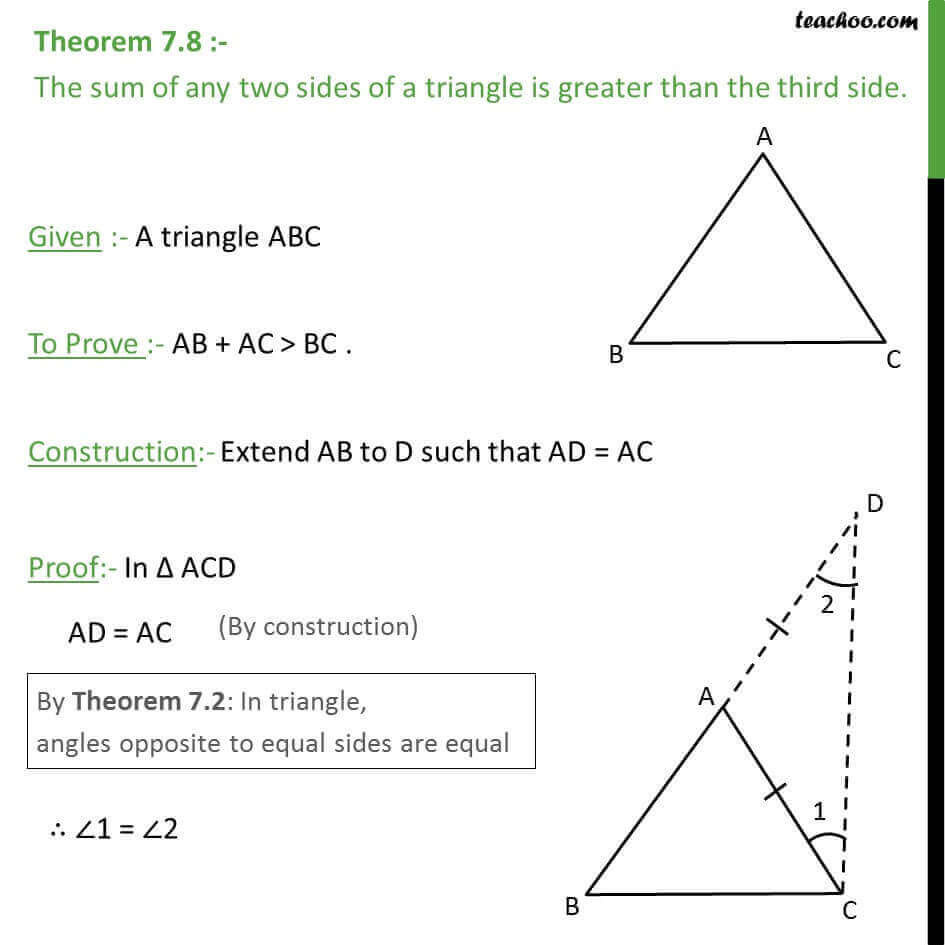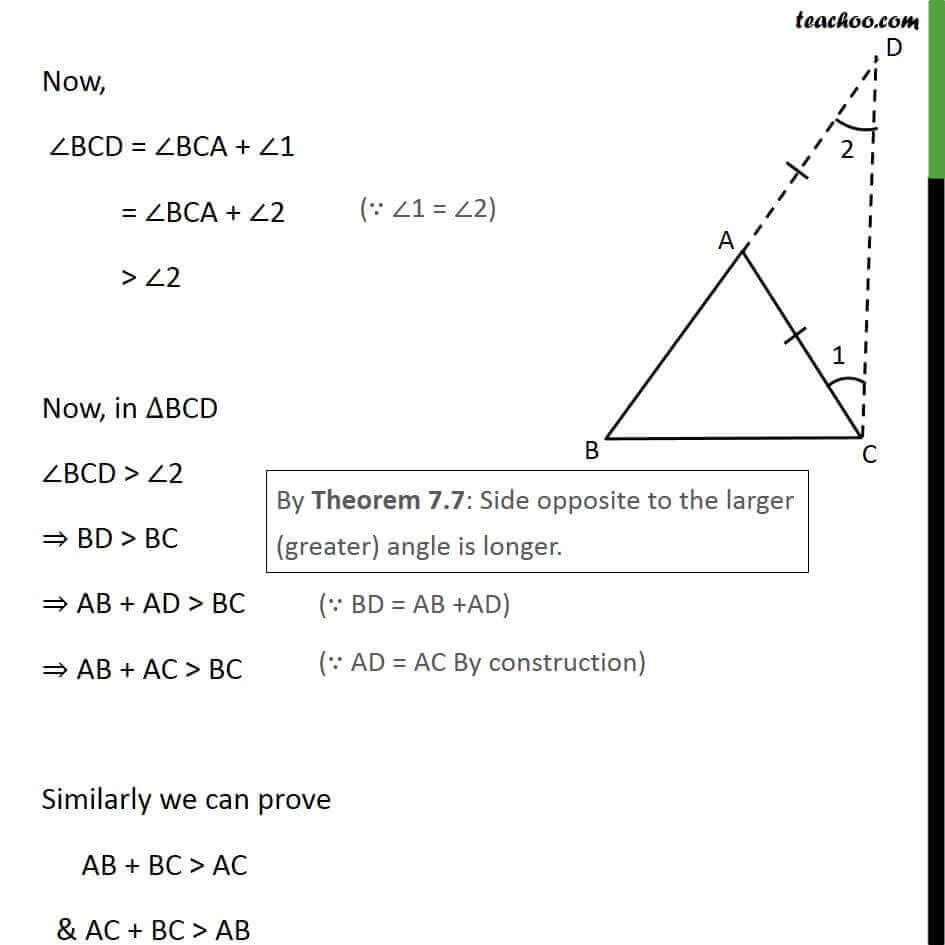1. Chapter 7 Class 9 Triangles (Term 1)
2. Serial order wise
3. Theorems

Transcript

Theorem 7.8 :- The sum of any two sides of a triangle is greater than the third side. Given :- A triangle ABC To Prove :- AB + AC > BC . Construction:- Extend AB to D such that AD = AC Proof:- In Δ ACD AD = AC ∴ ∠1 = ∠2 Now, ∠BCD = ∠BCA + ∠1 = ∠BCA + ∠2 > ∠2 Now, in ∆BCD ∠BCD > ∠2 ⇒ BD > BC ⇒ AB + AD > BC ⇒ AB + AC > BC Similarly we can prove AB + BC > AC & AC + BC > AB Hence Proved

Theorems

Chapter 7 Class 9 Triangles (Term 1)
Serial order wise